# What is the bus stop method?

The bus stop method is a simple way to divide. Children will usually be taught this method in year 5 or 6. It is sometimes known as short division or short-hand division.

Top Tip; it is SO much easier to learn to divide if your child knows their times tables.

### Let us show you how it’s done;

If we wanted to work out 468 ÷ 4.

1. Set out the numbers like this;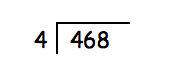Work out how many 4’s go into 4 (answer 1).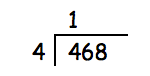1. Work out how many 4’s go into 6 (answer 1 remainder 2).

Place the answer (1) directly above the 6.

Carry the remainder (2) and place it above the next digit 8 to make the number 28.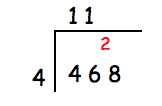1. Work out how many 4’s go into 28 (answer 7).

Place the answer (7) directly above the 28.

You can then clearly see that 468 ÷ 4 = 117.

If there is anything left over from the final division, this becomes your remainder.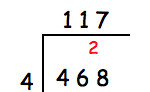## Bus stop method for two-or-three digit numbers

If you want to divide a two or three digit number, the bus stop method can also be used. Here’s an example;

If we wanted to work out 468 ÷ 42.

1. Set out the numbers like this;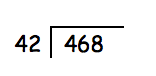1. Work out how many 42’s go into 46 (answer 1 remainder 4)

Carry the remainder (4) and place it above the next digit 8 to make the number 48.1. Work out how many 42’s go into 48 (answer 1 remainder 6).

Place the answer (1) directly above the 48.

You can then clearly see that 468 ÷ 42 = 11 remainder 6.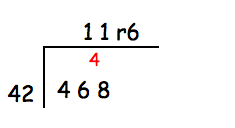# Bus stop method hacks

1. Learning your times tables is the best tip we can give you for division!
2. Try to memorise which numbers are likely to be divisible by certain numbers, for example, if the units contain a 5 or a 0, your number is likely to divide exactly into 5.
3. Estimate! We cannot stress how important this is. Look carefully at the calculation you are doing. Guess an approximate answer before you begin. For example, 468 ÷ 42 is going to be somewhere near 10, we know this because 10 x 42 is 420 and 468 isn’t that far off. Similarly, 468 ÷ 4 is likely to be somewhere near 110 because we know that 11 x 4 = 44 so 110 x 4 is 440.
4. Once you get your answer work it backwards to check, so if you think that 468 ÷ 42 = 11 r6. Try 11 x 42 + 6.
5. Make sure you write you calculation out carefully, stick to the correct columns (hundreds, tens and units). If necessary use squared paper to help you keep your digits in line.
6. If you get stuck in your head, try to imagine the division in ‘real life’ tell yourself a story. For example, 468 children were going on a school trip to the museum. Each coach could only fit 42 children. How many coaches would the school need? How many children would be left over to fit in the minibus?

# Worksheets and Practice

Division can be entertaining and once you’ve got to grips with it - you can use your new found knowledge to do lots of cool stuff! Whilst you’re learning though it’s always good to practice.

We have loads of great worksheets on division, and in particular, the bus stop method. We’ve listed a few of the most relevant here:

Year 4 – Know your division

Year 6 – Long division problems.

Year 6 – Formal long division

Year 6 – Written division calculations.

# Further Learning

If you enjoy learning division and want to give yourself a challenge, why not try some of the puzzles and problems set by the NRich team from the University of Cambridge?

Here are a selection of puzzles and problems linked the bus stop method and division to really get you thinking

https://nrich.maths.org/public/search.php?search=bus+stop+method

## Wait, don’t go! Keep Learning with EdPlace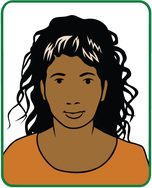###### AUTHOR, MS. ALISON – PRIMARY MATHS TEACHER.